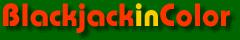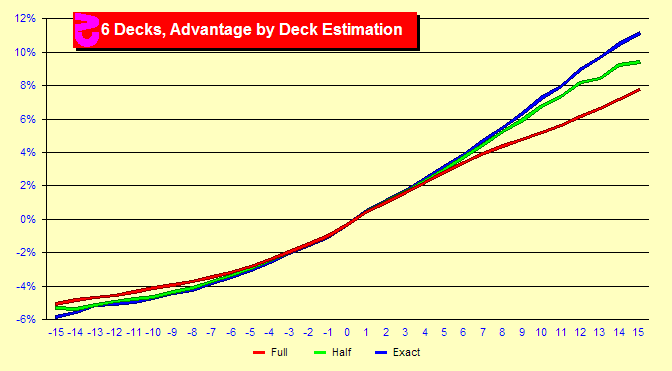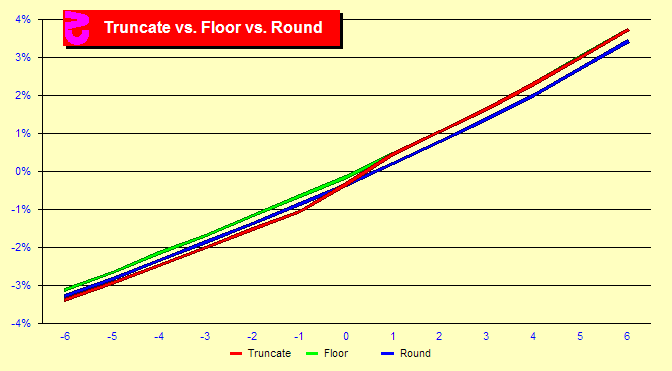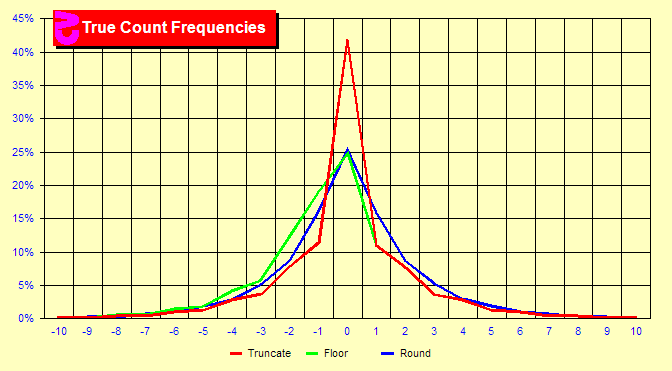# Effect of Blackjack True Count Methods

###How do different manners of True Counting differ?

There exist different methods of calculating a true count. (This page will not be of interest to running-count players.) You can estimate the remaining decks in different manners, round the results differently or include or exclude cards on the table among other variations. We will look at a couple of these variations here.

First, we must estimate the number of remaining cards. This chart shows three levels of accuracy in this estimate: remaining cards estimated to the nearest full decks, half decks or exact cards. All data are based on six decks. The blue line indicates an exact calculation of cards in the discard tray (not counting the cards on the table). As you can see the higher the accuracy, the higher the advantage at high counts. These counts generally occur late in the shoe where accuracy is more important. However, the important counts are +1 through +5 as this is where most of the money is bet. At these counts there is not a large difference. Therefore the overall gain in better accuracy is not that high unless the penetration is very deep. Charts on overall gain by penetration are available in later pages of this site.

###What about division rounding methods?

Another area of variability in true count calculation is the method of integerization after dividing the running count by remaining decks. Nearly all Blackjack card counters use integer true counts. When you divide 5 by 3, how do you turn this into an integer? (Most Blackjack books give easy examples like 6/3 and fail to touch on the question.) Some people round to the nearest integer (rounding), some always round down (flooring) and some round towards zero (truncation). Truncation is a bit less accurate because it greatly increases the number of zero counts. This reduces the resolution of the count. Rounding and flooring have about the same overall accuracy. As the chart shows, flooring and truncating have the same advantages at positive counts because they both round down at positive counts. But this changes below the zero count since truncation now rounds up. This means that truncated counts below zero will have a lower advantage. See the follwing graph for the details.

###How does this affect count frequencies?

If you integerize True Counts in different manners, the frequencies of those true counts will change. This chart provides the frequencies for the three methods of integerization. The green and red lines (flooring and truncation) are identical for counts of +1 and higher. The red line indicates a very large percentage (42%) of counts of zero for truncation. This is because all counts between -.999 and .999 will truncate to zero. Truncation is a bit inferior due to this large peak at TC zero. When you have so many hands all identified as a count of zero, the count is less precise and an index of zero less valuable. However, the overall effect on advantage is not high.

### Sim details

• Six decks, S17, DAS, LS, 4.81/6, Hi-Lo max indexes, trunc, half-deck
• Six decks, S17, DAS, LS, 4.81/6, Hi-Lo max indexes, trunc, full-deck
• Six decks, S17, DAS, LS, 4.81/6, Hi-Lo max indexes, trunc, exact cards
• Six decks, S17, DAS, LS, 4.81/6, Hi-Lo max indexes, round, half-deck
• Six decks, S17, DAS, LS, 4.81/6, Hi-Lo max indexes, floor, half-deck
• Ten billion rounds for six and two decks, five billion rounds for single-deck